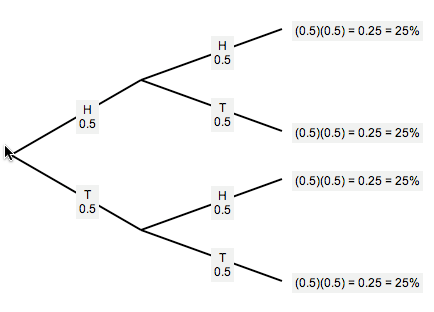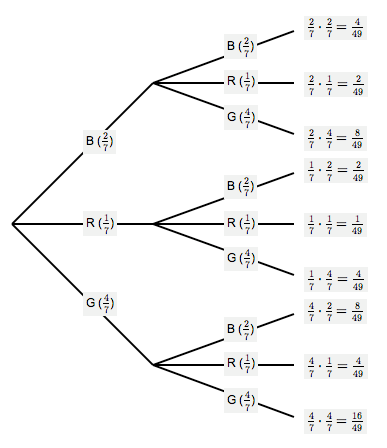﻿ Probability Tree Diagrams
PROBABILITY TREE DIAGRAMS
by Dr. Carol JVF Burns (website creator)
Follow along with the highlighted text while you listen!

A tree diagram represents the outcomes from a multi-step experiment.

A sample tree diagram is shown below.
Repeatedly click the button to get many more (randomly-generated) samples!

TWO-STEP PROBABILITY TREE DIAGRAMS

A multi-step experiment has more than one step.
For example, here are some two-step experiments:

• flip a coin and record Head or Tail (first step), then do it again (second step)
• choose a ball from an urn and record its color, then do it again
• flip a coin and record Head or Tail, then choose a ball from an urn and record its color

The branches emanating from any point on a tree diagram must have probabilities that sum to  1 .
In the sample tree diagram above (initial configuration), we have:

• $0.7 + 0.3 = 1$
• $0.2 + 0.7 + 0.1 = 1$
• $0.4 + 0.5 + 0.1 = 1$

To find the probability of any path, multiply the probabilities on the corresponding branches.
In the sample tree diagram above (initial configuration), there are six paths (going from top to bottom):

• $(0.7)(0.2) = 0.14$
• $(0.7)(0.7) = 0.49$
• $(0.7)(0.1) = 0.07$
• $(0.3)(0.4) = 0.12$
• $(0.3)(0.5) = 0.15$
• $(0.3)(0.1) = 0.03$
Notice that:   $(0.14 + 0.49 + 0.07 + 0.12 + 0.15 + 0.03) = 1$
Always do this check on your own probability tree diagrams!

EXAMPLES:
 TWO FLIPS OF A FAIR COIN Consider the two-step experiment ‘two flips of a fair coin’. Let   H   denote Head, and   T   denote Tail. Note that the sample space for this experiment is: $\,S = \{\text{HH},\text{HT},\text{TH},\text{TT}\,\}\,$ The probability tree diagram is shown at right. From the tree diagram, we can easily compute various probabilities: $\cssId{s41}{P(\text{both heads})} \cssId{s42}{= P(\text{HH})} \cssId{s43}{= (0.5)(0.5)} \cssId{s44}{= 0.25}$ $\cssId{s45}{P(\text{both tails})} \cssId{s46}{= P(\text{TT})} \cssId{s47}{= (0.5)(0.5)} \cssId{s48}{= 0.25}$ $\cssId{s49}{P(\text{no heads})} \cssId{s50}{= P(\text{TT})} \cssId{s51}{= 0.25}$ $P(\text{at least one head})$     $= P(\text{HH or HT or TH})$     $= P(\text{HH}) + P(\text{HT}) + P(\text{TH})$     $= 0.25 + 0.25 + 0.25 = 0.75$ (alternate method) $P(\text{at least one head})$     $= P(\text{not TT})$     $= 1 - P(\text{TT})$     $= 1 - 0.25 = 0.75$ $P(\text{exactly one head})$     $= P(\text{HT or TH})$     $= P(\text{HT}) + P(\text{TH})$     $= 0.25 + 0.25 = 0.50$ TWO FLIPS OF A FAIR COINBALLS IN AN URN, WITH REPLACEMENT Suppose that an urn contains 2 black balls, 1 red ball, and 4 green balls. Two balls are chosen, with replacement, from the urn. That is, after the first ball is chosen and its color is recorded, it is returned to the urn. Assume that all balls are equally likely to be chosen. Note that the sample space for this experiment is: $S = \{\text{BB},\text{BR},\text{BG},\text{RB},\text{RR},\text{RG},\text{GB},\text{GR},\text{GG}\}$ The probability tree diagram is shown at right. From the tree diagram, we can easily compute various probabilities: $\displaystyle \cssId{s75}{P(\text{BB})} \cssId{s76}{= \frac27\cdot\frac27} \cssId{s77}{= \frac4{49}}$ $\displaystyle \cssId{s78}{P(\text{RR})} \cssId{s79}{= \frac17\cdot\frac17} \cssId{s80}{= \frac1{49}}$ $\displaystyle \cssId{s81}{P(\text{GG})} \cssId{s82}{= \frac47\cdot\frac47} \cssId{s83}{= \frac{16}{49}}$ $P(\text{BB or RR})$     $= P(\text{BB}) + P(\text{RR})$     $= \frac4{49} + \frac1{49}$     $= \frac5{49}$ $P(\text{at least one black ball is drawn})$     $= P(\text{BB or BR or BG or RB or GB})$     $= \frac4{49} + \frac2{49} + \frac8{49} + \frac2{49} + \frac8{49}$     $= \frac{24}{49}$ $P(\text{at most one black ball is drawn})$     $= P(\text{exactly one black ball or no black balls})$     $= P(\text{all outcomes except BB})$     $= 1 - \frac4{49}$     $= \frac{45}{49}$ $P(\text{exactly one black ball is drawn})$     $= P(\text{BR or BG or RB or GB})$     $= \frac2{49} + \frac8{49} + \frac2{49} + \frac8{49}$     $= \frac{20}{49}$ $P(\text{two different color balls})$     $= P(\text{all outcomes except BB, RR, GG})$     $= 1 - \frac4{49} - \frac1{49} - \frac{16}{49}$     $= \frac{28}{49}$ BALLS IN AN URN, WITH REPLACEMENTBALLS IN AN URN, WITHOUT REPLACEMENT Suppose that an urn contains 2 black balls, 1 red ball, and 4 green balls. Two balls are chosen, without replacement, from the urn. That is, the first ball is chosen and its color is recorded. It is not returned to the urn. Then, the second ball is chosen. Assume that all balls are equally likely to be chosen. Note that the sample space for this experiment is: $S = \{\text{BB},\text{BR},\text{BG},\text{RB},\text{RG},\text{GB},\text{GR},\text{GG}\}$ There is no ‘RR’ in the sample space, since if a red ball is chosen on the first draw, then there are no red balls remaining in the urn. The probability tree diagram is shown at right. From the tree diagram, we can easily compute various probabilities: $\displaystyle \cssId{s119}{P(\text{BB})} \cssId{s120}{= \frac27 \cdot \frac16} \cssId{s121}{= \frac2{42}} \cssId{s122}{= \frac{1}{21}}$ $P(\text{RR}) = 0$ $\displaystyle \cssId{s124}{P(\text{GG})} \cssId{s125}{= \frac47 \cdot \frac36} \cssId{s126}{= \frac{12}{42}} \cssId{s127}{= \frac{2}{7}}$ $P(\text{the same color is drawn twice})$     $= P(\text{BB or GG})$     $= \frac1{21} + \frac2{7} = \frac1{21} + \frac6{21}$     $= \frac{7}{21} = \frac13$ $\displaystyle \cssId{s132}{P(\text{BR})} \cssId{s133}{= \frac27 \cdot \frac16} \cssId{s134}{= \frac2{42}} \cssId{s135}{= \frac{1}{21}}$ $\displaystyle \cssId{s136}{P(\text{RB})} \cssId{s137}{= \frac17 \cdot \frac26} \cssId{s138}{= \frac2{42}} \cssId{s139}{= \frac{1}{21}}$ $P(\text{a black ball and a red ball are drawn})$     $= P(\text{BR or RB})$     $= \frac1{21} + \frac1{21}$     $= \frac{2}{21}$ BALLS IN AN URN, WITHOUT REPLACEMENTMaster the ideas from this section

When you're done practicing, move on to:
Choosing Things: Does Order Matter?